SLVSDV8 July   2017

PRODUCTION DATA.

1. Features
2. Applications
3. Description
4. Revision History
5. Pin Configuration and Functions
6. Specifications
7. Detailed Description
1. 7.1 Overview
2. 7.2 Functional Block Diagram
3. 7.3 Feature Description
4. 7.4 Device Functional Modes
8. Application and Implementation
1. 8.1 Application Information
2. 8.2 Typical Application
1. 8.2.1 Design Requirements
2. 8.2.2 Detailed Design Procedure
3. 8.2.3 Application Curves
9. Power Supply Recommendations
10. 10Layout
11. 11Device and Documentation Support
12. 12Mechanical, Packaging, and Orderable Information

#### Package Options

Refer to the PDF data sheet for device specific package drawings

• RNV|18
• RNV|18

### 6.8 Typical Characteristics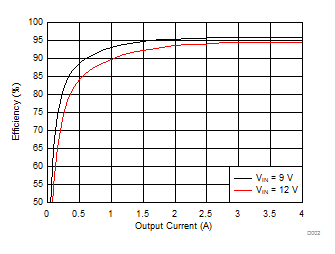VOUT = 5 V fSW = 800 kHz TA = 25 °C WE 744311220 L = 2.2 µH DCR = 11.4 mΩ
Figure 1. Efficiency for 5 V Output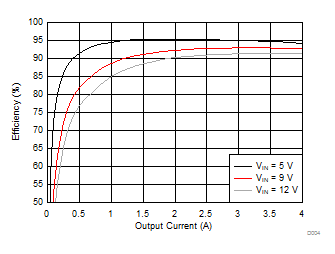VOUT = 2.5 V fSW = 700 kHz TA = 25 °C WE 744311220 L = 2.2 µH DCR = 11.4 mΩ
Figure 3. Efficiency for 2.5 V Output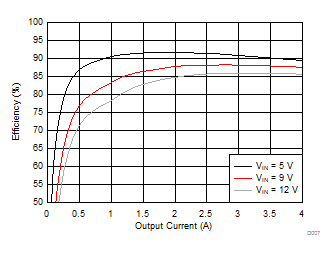VOUT = 1.2 V fSW = 600 kHz TA = 25 °C WE 74438357012 L = 1.2 µH DCR = 13.4 mΩ
Figure 5. Efficiency for 1.2 V Output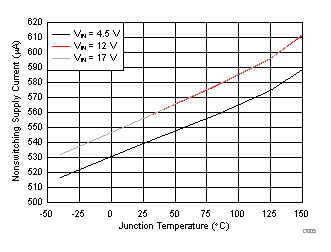V(EN) = 5 V V(FB) = 0.8 V
Figure 7. VIN Pin Nonswitching Supply Current vs Junction TemperatureFigure 9. EN Pin Voltage Threshold vs Junction Temperature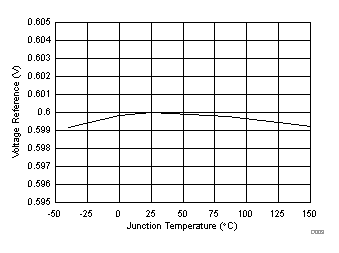Figure 11. Regulated FB Voltage vs Junction Temperature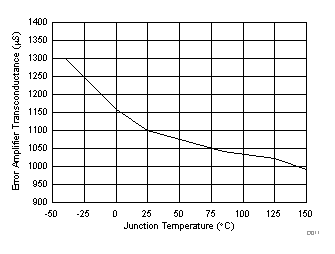Figure 13. Error Amplifier Transconductance vs Junction Temperature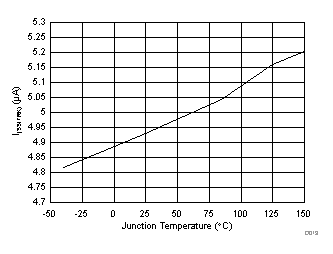Figure 15. SS/TRK Current vs Junction Temperature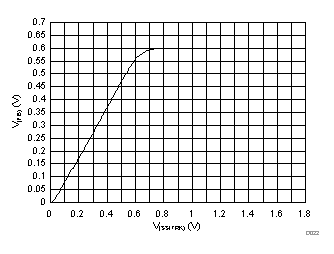Figure 17. FB voltage vs SS/TRK Voltage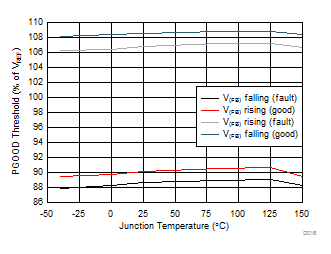Figure 19. PGOOD Thresholds vs Junction Temperature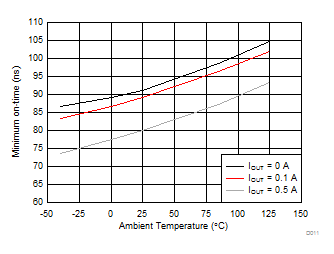VIN = 12 V L = 1.5 µH
Figure 21. Minimum on-time vs Ambient TemperatureR(RT/CLK) = 30.1 kΩ
Figure 23. Switching Frequency vs Junction Temperature (1600 kHz)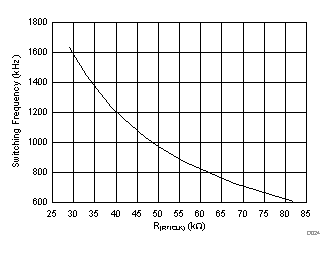Figure 25. Switching Frequency vs RT/CLK Resistor (High Range)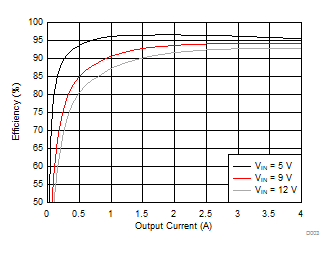VOUT = 3.3 V fSW = 700 kHz TA = 25 °C WE 744311220 L = 2.2 µH DCR = 11.4 mΩ
Figure 2. Efficiency for 3.3 V OutputVOUT = 1.5 V fSW = 700 kHz TA = 25 °C WE 74438357018 L = 1.8 µH DCR = 18 mΩ
Figure 4. Efficiency for 1.5 V Output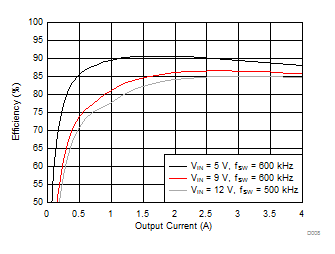VOUT = 1.0 V TA = 25 °C TA = 25 °C WE 74438357012 L = 1.2 µH DCR = 13.4 mΩ
Figure 6. Efficiency for 1.0 V Output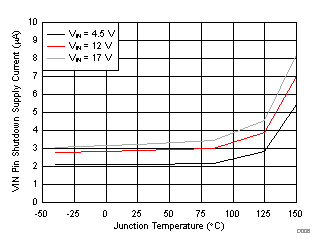V(EN) = 0.4 V
Figure 8. VIN Pin Shutdown Current vs Junction Temperature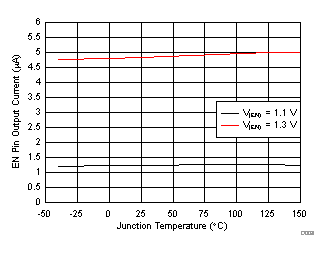Figure 10. EN Pin Current vs Junction Temperature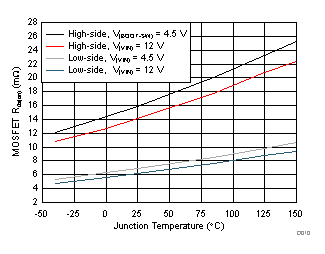Figure 12. MOSFET Rds(on) vs Junction Temperature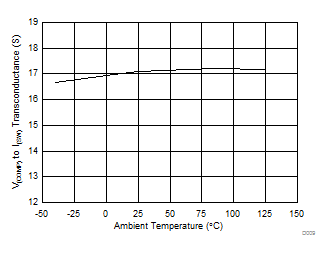Figure 14. COMP to SW Transconductance vs Junction TemperatureV(SS/TRK) = 0.4 V
Figure 16. SS/TRK to FB Offset vs Junction Temperature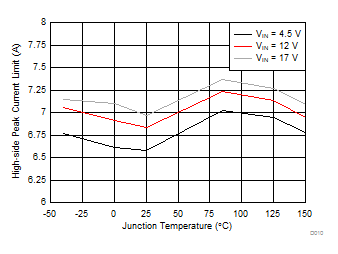Figure 18. High-side Peak Current Limit vs Junction TemperatureV(FB) = 0.6 V V(PGOOD) = 5 V
Figure 20. PGOOD Leakage Current vs Junction Temperature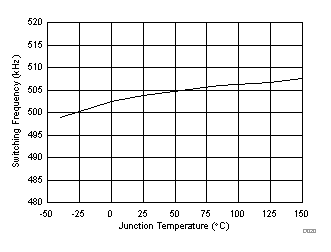R(RT/CLK) = 100 kΩ
Figure 22. Switching Frequency vs Junction Temperature (500 kHz)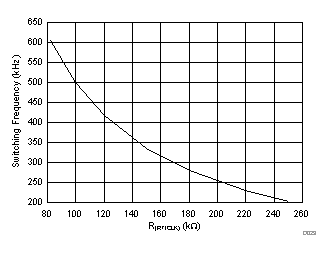Figure 24. Switching Frequency vs RT/CLK Resistor (Low Range)